New update is available. Click here to update.

# C program to delete vowels from a string

## Introduction

The vowels 'A', 'E', 'I', 'O', and 'U' are five vowels out of 26 characters in the English alphabet letters, and we will learn how to create a program to delete them in this article. Because C programming is case sensitive, lowercase and uppercase characters are treated differently, we must check for both cases before removing the vowels from a string.Also Read, Binary to Hex Converter

## Problem Statement

Write a program that takes a single string as input and removes the vowels a, e, i, o, and u.

### What will our vowel-delete program do?

• The input to our program will be a string.
• We'll cycle through the string, checking whether each character is a vowel or not.
• Shift the right element to the left if the vowel is found. Our difficulty will be solved as a result of this.

## Naive Approach

The idea is to iterate over every character of a string and check whether it is a vowel or not. If vowel, then delete the character from the string and move into the next element.

### Dry Run

For a better understanding of the approach shown by a figure.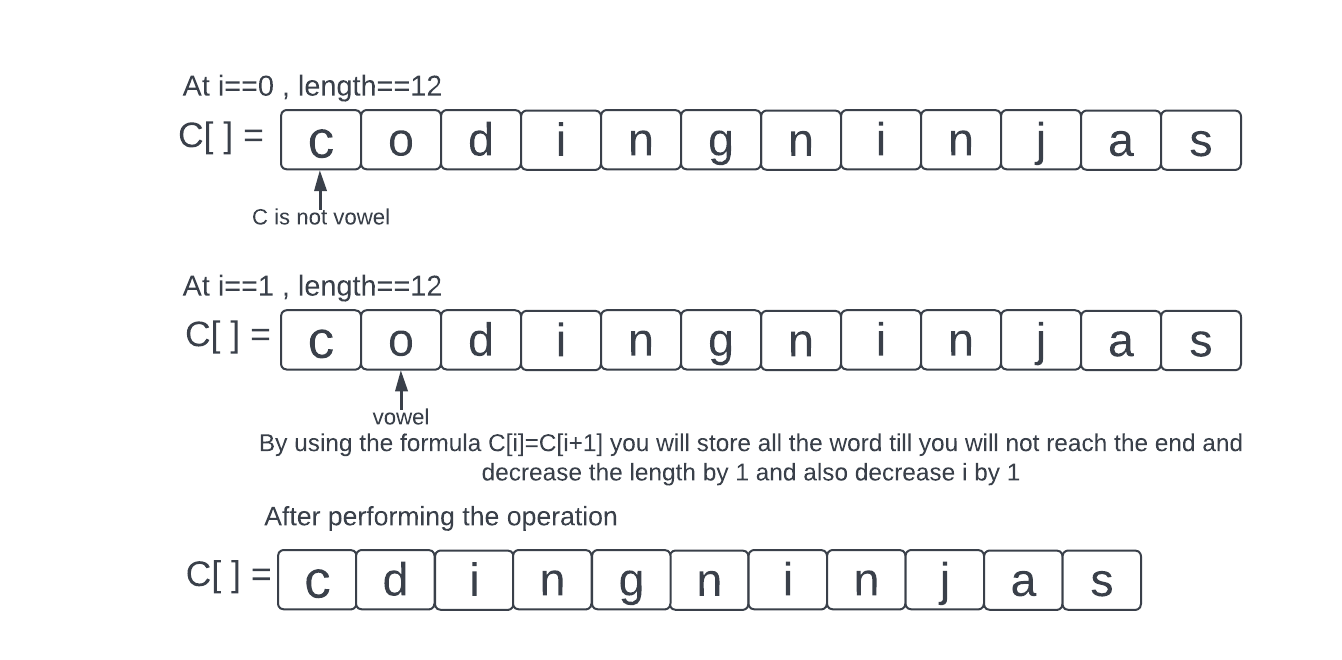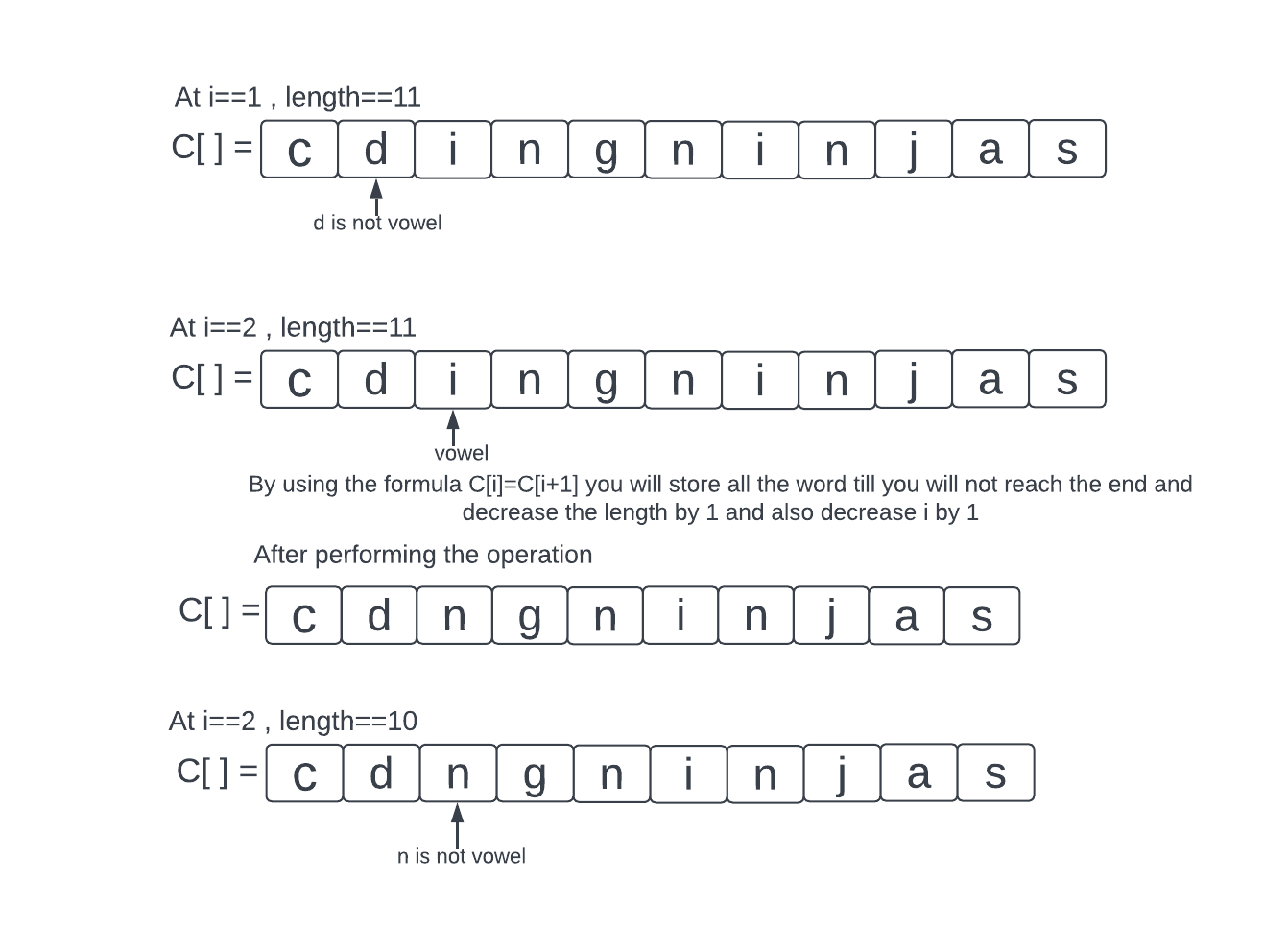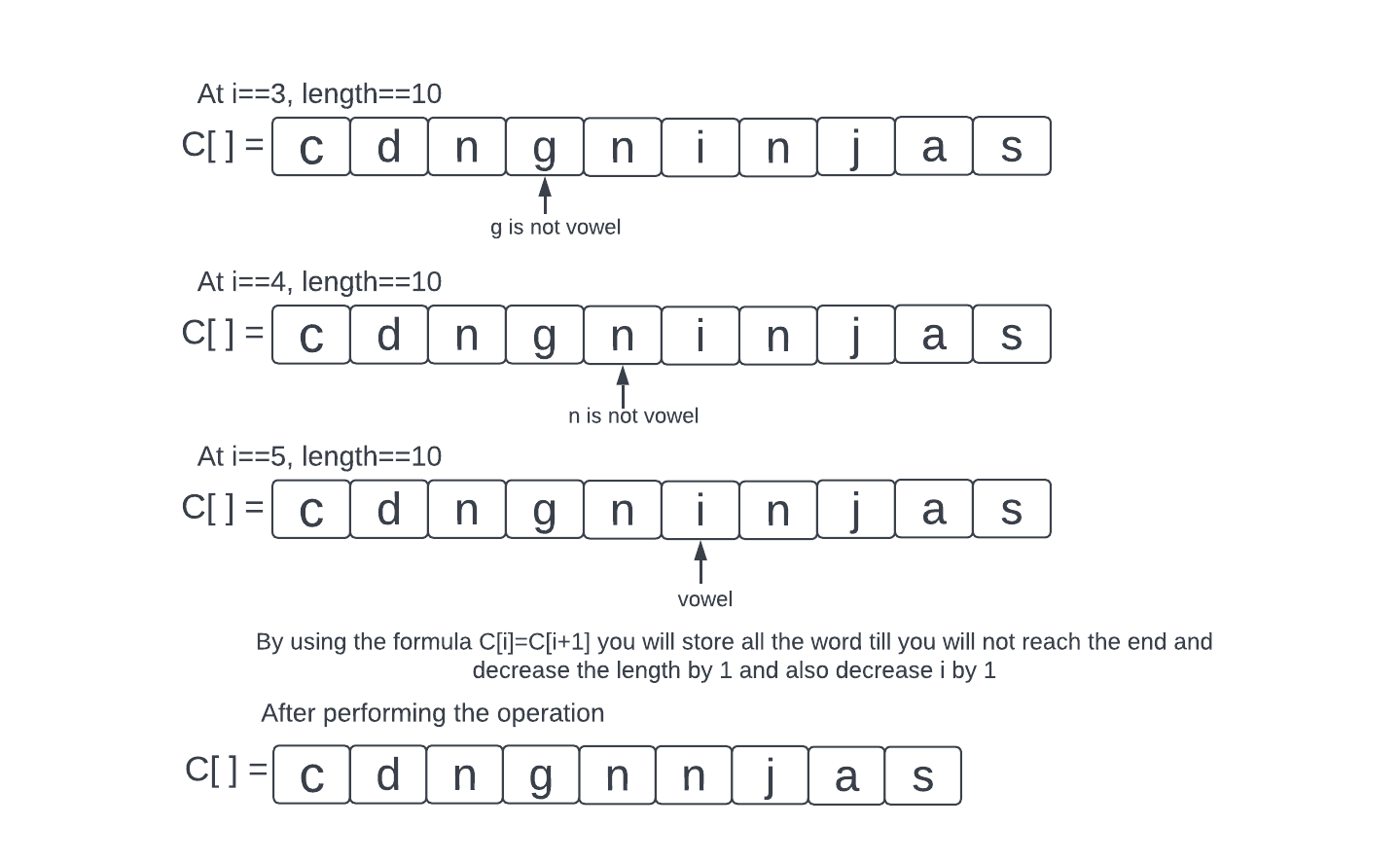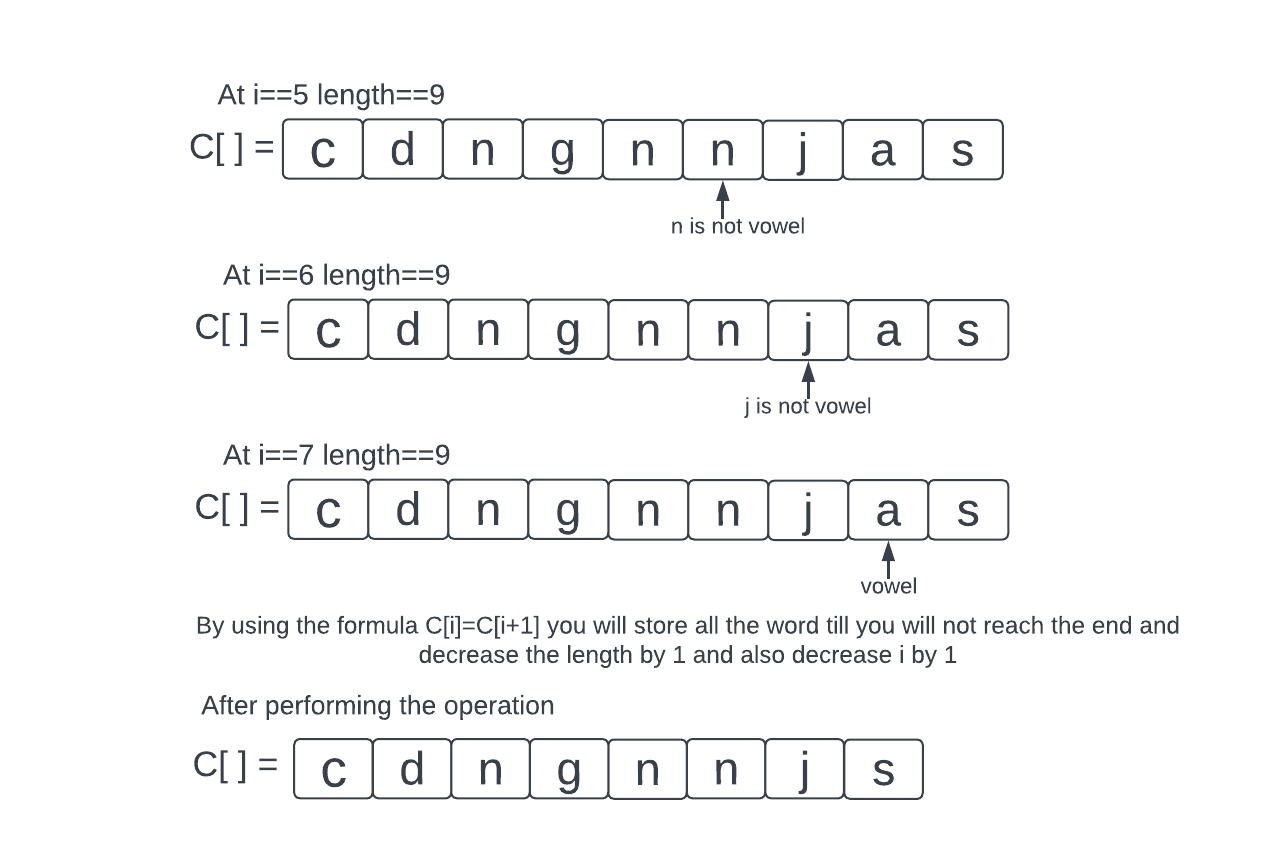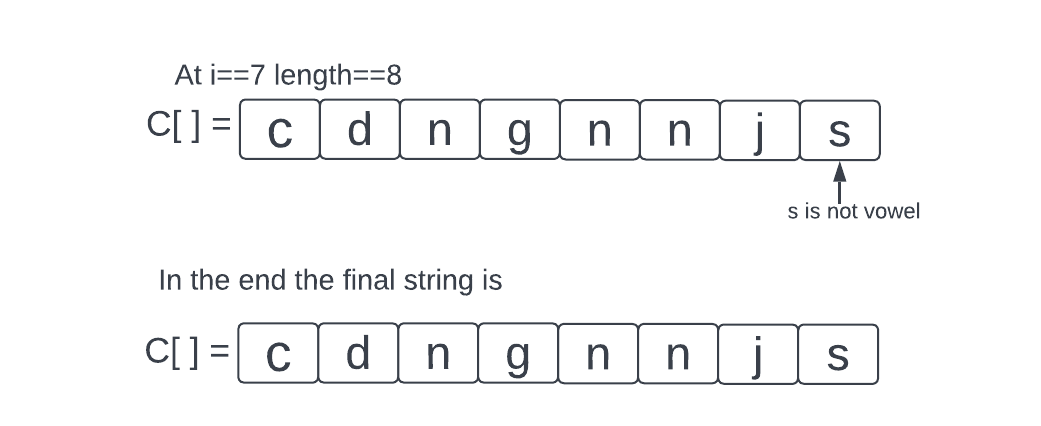Now, let us discuss the algorithm:

### Algorithm

1. Set up the variables.
2. Accept the data.
3. Set up the loop.
4. Check the vowels and remove them.
5. Using another for loop, store the string that is devoid of vowels.
6. Both loops should be ended.
7. Remove all vowels from the string and print it.

### Implementation

``````#include <stdio.h>
#include <stdlib.h>
#include<string.h>
void solve()
{
// Initializing variable.
char c;
int i, j, length = 0;

// Accepting input.
printf("Enter a string : ");
printf("\n");
// gets(c);
scanf("%s", c);

length = strlen(c);

for (i = 0; i < length; i++) {
// Checking vowels.
if (c[i] == 'A' || c[i] == 'E' || c[i] == 'I' || c[i] == 'O' || c[i] == 'U' ||
c[i] == 'a' || c[i] == 'e' || c[i] == 'i' || c[i] == 'o' || c[i] == 'u' ) {
// Deleting vowels.
for (j = i; j < length; j++) {
// Storing string without vowels.
c[j] = c[j + 1];
}
i--;
length--;
}
c[length + 1] = '\0';
}
printf("thre final string will be after deletion  : %s", c);

}
// main function starts.
int main()
{
solve();
return 0;
}``````

OutputTime complexity: O(n^2), where n is the length of the string.

Space complexity: Constant space as we are modifying the input array.

## Another Approach

In the previous approach, the time complexity is O(n^2). So we need to find another approach that reduces the time complexity. For that, we take another variable (t) and traverse the given string by a loop and by using function call check if the character is not vowel then push that character in that variable(t) and finally we copy that data in the original variable and print them. Hence, through this, we can reduce the time complexity to O(n). Now let’s see the algorithm and the implementation of this approach.

### Algorithm

1. Set up the variables.
2. Accept the data.
3. Set up the loop.
4. Check the vowels.
5. If vowels are present then don’t copy that data(vowel) into another variable.
6. If that data is not vowel then copy that data into another variable.
7. Finally copy the data in the original variable and then print them.

### Implementation

``````#include <stdio.h>
#include <string.h>
int check_vowel(char);
int main()
{
char c;
char t;
int s = 0;
int  d = 0;
printf("Enter a string \n");
gets(c);
for (s = 0; c[s] != '\0'; s++) {
if (check_vowel(c[s]) == 0) {
t[d] = c[s];
d++;
}
}
t[d] = '\0';
strcpy(c, t);
printf("The final string will be after deletion: %s\n", c);
return 0;
}
int check_vowel(char t)
{
if (t == 'a' || t == 'e' || t == 'i' || t =='o' || t == 'u' || t == 'A' ||  t == 'E' || t == 'I' ||  t=='O' || t == 'U')
return 1;
return 0;
}``````

Output

Implement this code on online compiler for better practice.

Time Complexity: O(n), where “n” is the length of the string. As the loop runs the n number of times.

Space Complexity: O(n), where “n” is the length of the string. As the extra space required is equal to the length of the string.

### How do you delete a letter in a string C?

RemoveChar() is a function that takes a string array address as an input and a character to delete from a string as an output. Our reasoning is now written in the removeChar(char *str, char charToRemove) method.

### What are vowels called?

A vowel is defined as a letter that represents a speech sound made with the vocal tract open, such as the letters A, E, I, O, and U.

### How do you check if a string has a vowel in C?

We can print the locating and character if the character matches. NOTE: To read an input string, such as Hello World, we must utilize the scanf function with the expression scanf(“%[^\n]s”, str); – notice the [^\n]. It will read the string, including space.

### How do I remove a character from a string?

We can use replace() to replace a specific character. If we wish to get removal of a certain character, we can do so by replacing it with an empty string. All occurrences of the specified character will be replaced by the str. replace() function.

### What is the Memmove function in C?

The memmove() function copies the number of bytes from source to dest. As if src were first put into a temporary array, this function permits copying between objects that may overlap—the return value.

## Conclusion

In this article, we see the implementation of the  C program to delete vowels from a string. We had also seen the output of the written program on some random input.

Transpose of a Matrix in C

C Program to count the length of string without using any library function

Armstrong Number in C

Program to convert Fahrenheit to Celsius

Check out this problem - Longest String Chain

Please refer to our guided paths on Codestudio to learn more about DSA, Competitive Programming, JavaScript, and System Design, etc. And also, enroll in our courses and refer to the mock test and problems available. Have a look at the interview experiences and interview bundle for placement preparations.Please upvote our blog to help other ninjas grow.

Happy Learning!